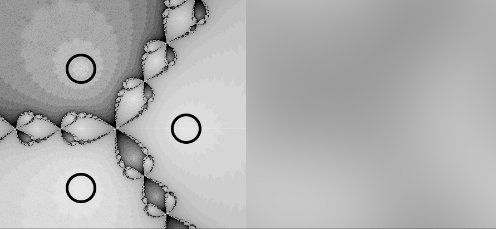ねこでじ（Nekodigi)

# 【Processing】Implement 2D FFT and 2D IFFT and Gaussian blur with them. (Cooley-Tukey Algorithm)

### AbstractI found that I could extend the FFT(Cooley Tukey Algorithm) easily to two dimensions so I implemented it immediately.I can convert an image to frequency data and I can get the original image from the frequency data with IFFT. It is often used for data compression and blur.Usually, the calculation time for Gaussian blur increases exponentially depending on its size. However, it takes the same time if even it's big if I use FFT.

### How it works

#### 1D FFT and IFFT

This algorithm based on the 1D FFT and IFFT (Cooley-Tukey algorithm). Please refer to these sites and codes for that.
Cooley–Tukey FFT algorithm - Wikipedia
github.com
for IFFT.
Cooley–Tukey Fast Fourier Transform algorithm - Recursive Divide and Conquer implementation in C++ · GitHub

#### 2D FFT and IFFT using 1D ones.

Roughly speaking, we can get 2D FFT by calculating FFT for each direction. I implement it by doing 1D FFT for each row and for each column.

#### Gaussian blur

I Fourier transforms the original image. Then I make a Gaussian kernel and Fourier transforms it. I multiply and inverse Fourier transforms them and I can get a Gaussian blurred image. Finally, shift image by FFT shift we can get the final result.
based on this site
Simple image blur by convolution with a Gaussian kernel — Scipy lecture notes

### Source code

I added it as Cooley Tukey 2D FFT.
github.com